Next: 3.3 Effect of Strain Up: 3. Strain Effects on Previous: 3.1 Theory of Elasticity

Subsections

# 3.2 Structure of Relaxed Si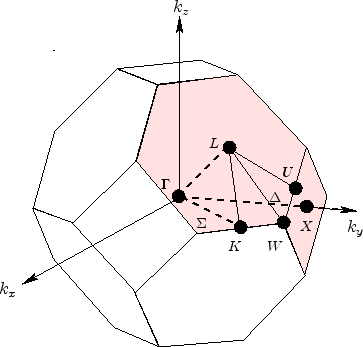Figure 3.4: (a) Structure of the fcc Si crystal lattice.(b) Brillouin zone of a fcc lattice with the notation for special symmetry direction and points.

The crystal structure of Si is classified under the diamond structure and consists of two inter-penetrating face centered cubic (fcc) lattices [Davies98]. The structure can be visualized as a tetrahedron with four vertices of the first fcc lattice at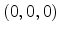,,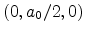and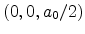and an additional atom added to the center of this tetrahedron. The additional atom, also known as motif, is thus displaced by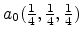with respect to the original fcc lattice. The lattice structure is shown in Fig. 3.4a. Here,nm denotes the lattice constant of unstrained Si. It can be seen that the structure has 8 atoms per unit cell. The distance of the additional atom to its four nearest neighbors is.

The Bravais lattice vectors for Si are given by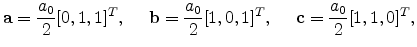(3.32)

from which the reciprocal lattice vectors can be obtained using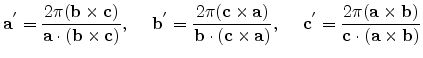(3.33)

to give(3.34)

These reciprocal lattice vectors correspond to a body centered cubic (bcc) lattice in the reciprocal space. The Wigner-Seitz cell of this bcc lattice is the first Brillouin zone (BZ). This primitive unit cell reflects the full symmetry of the lattice and is equivalent to the cell obtained by taking all points that are closer to the centre of the cell than to any other lattice point.

The first Brillouin zone has the shape of a truncated octahedron. It can be visualized as a set of eight hexagonal planes halfway between the centre of the cell and the lattice points at the corner, and six square planes halfway to the lattice points in the center of the next cell. The Brillouin zone is shown in Fig. 3.4b with the points and directions of high-symmetry marked using Greek letters and Roman letters for points on the surface. Table 3.3 summarizes these symmetry points and directions. These points and directions are of importance for interpreting the band structure plots.

 Symmetric Points/ Directions Coordinate Remark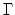(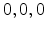) Origin of k space(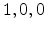) Middle of square faces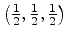Middle of hexagonal facesMiddle of edge shared by two hexagonsMiddle of edge shared by a hexagons and a squareMiddle of edge shared by two hexagons and a square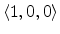Directed fromtoDirected fromtoDirected fromtoTable 3.3: Summary of the symmetry points and directions in the Brillouin zone.

Several symmetry operations can be performed on the diamond structure which leave the structure unchanged. Apart from translation, such operations can be categorized as rotation or inversion, or a combination, and are collectively known as symmetry operations. Due to the fcc lattice of the diamond structure there exist a total of 48 such independent symmetry operations. This symmetry of the lattice in real space is also reflected in the first BZ. The lowering of symmetry of the fcc structure due to strain can distort it to some of the 14 Bravais lattice forms, such as the tetragonal, orthorhombic or trigonal lattice. The new lattice structure formed has different symmetry properties which can have pronounced consequences on the band structure. Numerical methods for calculating the band structure allow the prediction of several important electronic and optical properties.

## 3.2.1 Band StructureFigure 3.5: (a) The band structure of Si as calculated using the non-local pseudopotential method in [Chelikowsky76]. (b) The valence band structure consisting of the degenerate heavy-hole (HH), the light-hole (LH) bands together with the split-off band.

The numerical band structure of unstrained Si calculated using the empirical pseudopotential method  [Chelikowsky76] is shown in Fig. 3.5. At, the highest energy band that is completely filled is denoted as the valence band. The next higher band is completely empty atand called the conduction band. The two bands are separated by a forbidden energy gap, known as the bandgap.

The principal conduction band minima of Si are located along the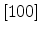,anddirections at a distance of about 85% from the-point to the X-points. The minima of the second conduction band touch the first conduction band at the X-points. This degeneracy of the two conduction bands can produce interesting effects on applying shear strain as discussed in Section 3.3.4. Close to the principal minima the band structure can be represented by ellipsoidal constant energy surfaces by assuming a parabolic energy dispersion relation, as shown in Fig. 3.6a. For valleys located along the  direction, the energy dispersion reads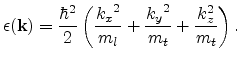(3.35)

where the wave vector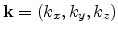. The constant energy surfaces are described by a longitudinal mass,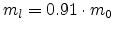, and a transversal mass,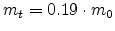. This anisotropy in the masses makes the transport in each valley anisotropic. The 6-fold degeneracy of the valleys arise due to the symmetry of the lattice along the,, anddirections. At low values of the electric field, the electrons occupy all the 6 valleys equally and thus the transport is isotropic.

Unlike the conduction band, the valence band structure looks highly anisotropic even in the unstrained case. The valence band maximum in Si is located at thepoint. Due to the degeneracy of the bands at thepoint, the energy surfaces develop into quartic surfaces of the form [Dresselhaus55](3.36)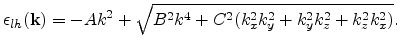(3.37)

Here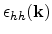and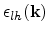denote the energies of the two degenerate bands and the parameters,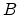anddenote the inverse band parameters. Due to the smaller energy values of thebands in comparison to the, the effective masses for theband is larger. Therefore, this band is referred to as the heavy hole (HH) band and theband as the light hole (LH) band. The shape of the constant energy surface for the LH and HH bands is warped or fluted. There is also a third band, the so called split-off band located about 44 meV below the HH/LH bands. A schematic plot of the constant energy surface for the HH band is shown in Fig. 3.6b.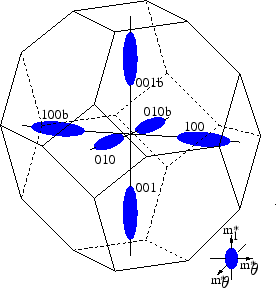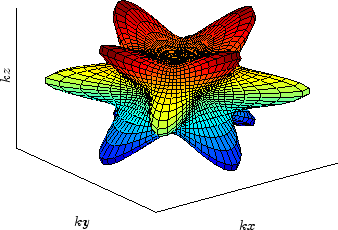Figure 3.6: Constant energy surface for unstrained Si . (a) Six-fold degenerate conduction band valleys located along thedirections. (b) Equi-energy surface of the heavy-hole valence band.

### 3.2.1.1 Band Structure Calculation Methods

The band structure of Si may be obtained using methods such as the tight binding approximation [Chaney71], the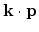method [Cardona66], and the empirical pseudopotential method [Chelikowsky76]. The tight binding approximation relies on wave functions of atomic orbitals and is generally suitable to describe the core atomic shells. It is however not a good choice for describing the conduction electrons due to their delocalized nature.

In themethod, the single-electron Schroedinger equation is expanded using Bloch's theorem, to yield aterm and an additional term parabolic into the original equation.(3.38)

Here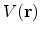is a local potential and the subscripts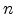and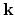refer to the band index and the wave vector, respectively, and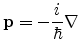is the momentum operator. The function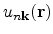is a periodic function having the periodicity of the lattice. Once the energy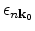and the periodic functionare known at some point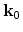, the complete band structure can be obtained using either degenerate or non-degenerate perturbation theory by treating the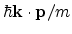terms as perturbation. The method serves as a good approximation for calculating the minimum of the conduction band and the valence bands in many group III-V and group IV semiconductors.

A very powerful tool for obtaining the complete band structure is the empirical pseudopotential method. In this method, the actual hard core ionic potential,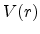, experienced by an electron is replaced by a soft pseudopotential,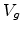. This also results in a modification of the true wave function,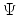, to a pseudo-function,, as shown in Fig. 3.7. The pseudopotential is found to depend on pseudopotential form factors which can be obtained by matching experimental results and are available for a large set of material systems [Yu03]. Knowing this potential, the Schroedinger equation gets modified to a so called pseudo wave equation, the solution of which delivers the energy dispersion relation.Next: 3.3 Effect of Strain Up: 3. Strain Effects on Previous: 3.1 Theory of Elasticity

S. Dhar: Analytical Mobility Modeling for Strained Silicon-Based Devices